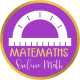Prism

A prism is a solid figure that has two parallel congruent sides that are called bases that are connected by the lateral faces that are parallelograms. There are both rectangular and triangular prisms.Surface area of a Prism

If we want to find the total surface of a prism we need to add twice the surface of the base plus the lateral surface.In order to find the surface of the base you only have to calculate the surface of a bidimensional shape.

The lateral surface is given by the perimeter of the base multiplied by the height:Inverse formulasVolume

To find the volume of a prism (it doesn’t matter if it is rectangular or triangular or else) we multiply the area of the base, called the base area S, by the height h.Inverse formulas:Exercises

1. A letter ‘T’ shape is made by sticking together 2 cuboids as shown in the diagram.

What is the total volume in cm³ and the surface area in cm2 of the letter ‘T’?2. Calculate the volume and the surface area of each of the cuboids below.3. A prism has a height of 32 cm. The base is a rhombus and the diagonals are one 3/4 of the other, their difference is 18 cm. Calculate the total surface area of the prism. Solution

4. A prism has a height of 978 cm. The base is a right angled trianglewith the hypotenuse 75 cm long; the hypotenuse is 25/7 of one of the legs. Calculate the total surface area. Solution

5. A prism has a right angled triangle as base. One leg is 27 cm long and the area of the triangle is 486 cm2 . The total surface area of the prism is 7776 cm2 . Calculate the volume and the height of the prism. Solution

6. Calculate the surface area and the volume of the following solids:7. The followimg image shows a prism whose base is a trapezium. Calculate the total surface area of the prism.8. The following image shows a prism whose base is a trapezium. Given that the total surface area of the prism is 126 cm2 , find the value of x.2 thoughts on “Prism”

1.Murray says:

I discovered your web site from Google and I need to say it was an excellent find.
Thanks!

Like

1.matemaths says:

I’m glad you liked it. I’m trying to upload new topics every week. Hope you’ll find what you are looking for. If not, just ask and I’ll do my best.

Like

This site uses Akismet to reduce spam. Learn how your comment data is processed.